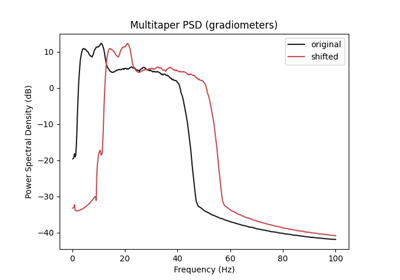# braindecode.augmentation.FrequencyShift#

class braindecode.augmentation.FrequencyShift(probability, sfreq, max_delta_freq=2, random_state=None)[source]#

Add a random shift in the frequency domain to all channels.

Note that here, the shift is the same for all channels of a single example.

Parameters
• probability (float) – Float setting the probability of applying the operation.

• sfreq (float) – Sampling frequency of the signals to be transformed.

• max_delta_freq (float | torch.Tensor, optional) – Maximum shift in Hz that can be sampled (in absolute value). Defaults to 2 (shift sampled between -2 and 2 Hz).

• random_state (int | numpy.random.Generator, optional) – Seed to be used to instantiate numpy random number generator instance. Defaults to None.

Methods

get_augmentation_params(*batch)[source]#

Return transform parameters.

Parameters
• X (tensor.Tensor) – The data.

• y (tensor.Tensor) – The labels.

Returns

params – Contains

• delta_freqfloat

The amplitude of the frequency shift (in Hz).

• sfreqfloat

Sampling frequency of the signals to be transformed.

Return type

dict

static operation(X, y, delta_freq, sfreq)#

Adds a shift in the frequency domain to all channels.

Note that here, the shift is the same for all channels of a single example.

Parameters
• X (torch.Tensor) – EEG input example or batch.

• y (torch.Tensor) – EEG labels for the example or batch.

• delta_freq (float) – The amplitude of the frequency shift (in Hz).

• sfreq (float) – Sampling frequency of the signals to be transformed.

Returns

• torch.Tensor – Transformed inputs.

• torch.Tensor – Transformed labels.

## Examples using `braindecode.augmentation.FrequencyShift`#Data Augmentation on BCIC IV 2a Dataset

Data Augmentation on BCIC IV 2a Dataset